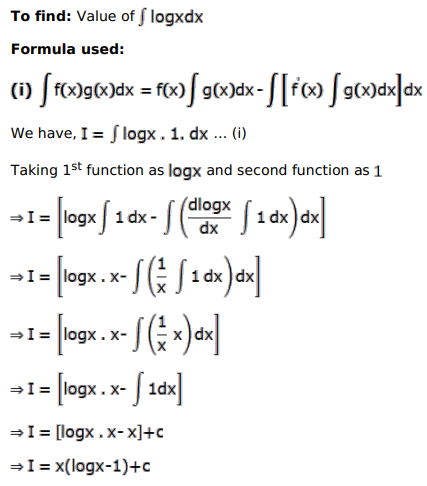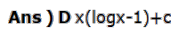# Solve this following

Question:

Mark $(\sqrt{)}$ against the correct answer in each of the following:

$\int \log x d x=?$

A. $\frac{1}{\mathrm{x}}+\mathrm{C}$

B. $\frac{1}{2}(\log x)^{2}+C$

C. $x(\log x+1)+C$

D. $x(\log x-1)+C$

Solution: